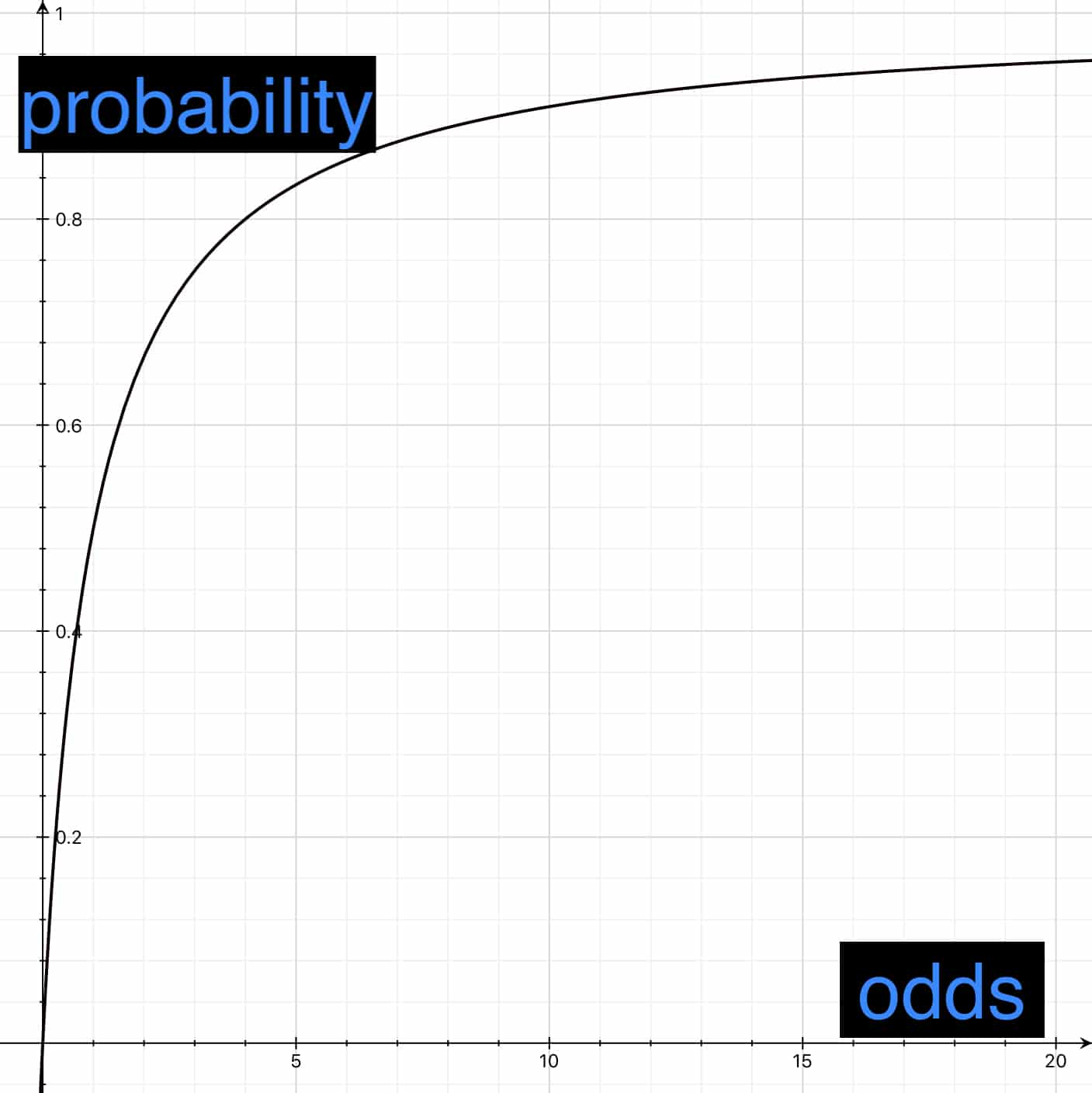## What is probability, what are odds, and how are the two related?

Probability is the chance that something will happen. It can be expressed as a fraction, a decimal, or a percent. If something is expected to succeed one time out of two, its probability is .

Odds compares the chance that a thing will happen with the chance that it will not. Odds is usually expressed as a ratio — e.g., . That means the same thing as ; the difference is just formatting. If something is expected to succeed one time out of two, that means one success for one failure, and its odds are 1:1.

In a statistics course, you may hear more about probability than you do about odds. In real life, you probably hear both: the probability of rain today and the odds that your horse will win. Statisticians use both probability and odds.

## How do odds and probability compare?

• If something is absolutely not going to happen, its odds and its probability are both zero.
• You can’t get less likely than absolutely not going to happen: Neither odds nor probability can be negative.
• If something is certain to happen:
• Its probability is one; that is, 100%.
• The chance that it will not happen is zero, so odds, the chance that it will happen v. the chance that it won’t, is infinite.

More specifically:

Say is the chance that something will happen and is the chance that it will not. Odds, the ratio of the chance that a thing will happen to the chance that it will not, is . Call odds C. Then .

It is certain that either a thing will happen or it will not; therefore , so .

In the equation , replace with . Then

That’s an expression for for a given .

Solve that for and you get:

That’s an expression for for a given .

This is how they compare graphically:Odds for a given probability: O=P/(1-P). As probability approaches one, odds increase without limit.Probability for given odds: P=O/(O+1). As odds increase, probability approaches one.

In the graph on the left, note that as probability increases toward 1, odds increases without limit. Probability always has a value between 0 and 1.

Odds, on the other hand, can increase without limit.

The table on the right shows odds for some probabilities:

 Probability Odds 0 0 0.01 1:99 0.10 1:9 0.25 1:3 0.50 1:1 0.75 3:1 0.90 9:1 0.99 99:1